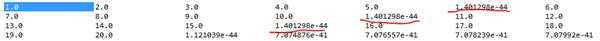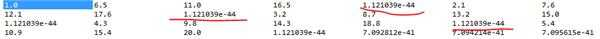﻿ malloc函数分配空间不连续问题 | Code Bye

# malloc函数分配空间不连续问题

8年前 (2015-10-22) 1520次浏览

```	M.mat = (float32**)malloc( row*sizeof(float32*) );
for(i=0;i<row;i++)
M.mat[i] = (float32*)malloc( col*sizeof(float32) );```

1,2,3,4,5
6,7,8,9,10
11,12,13,14,15
16,17,18,19,20LZ想说两次分配行数据的内存不连续吗？

```M.mat = (float32*)malloc( row*col*sizeof(float32));
for(i=0;i<row;i++) for (j=0;j<col;j++) M.mat[i*col+j] = 0.0;
//...
free(M.mat);```

```M.mat = (float32**)malloc( row*sizeof(float32*) );
float32 *buf = (float32 *)malloc(row*col*sizeof(float32));
for(i=0;i<row;i++)
M.mat[i] = buf + i * col;
```

LINUX 程序设计第四版
P218页

CodeBye 版权所有丨如未注明 , 均为原创丨本网站采用BY-NC-SA协议进行授权 , 转载请注明malloc函数分配空间不连续问题

[1034331897@qq.com]